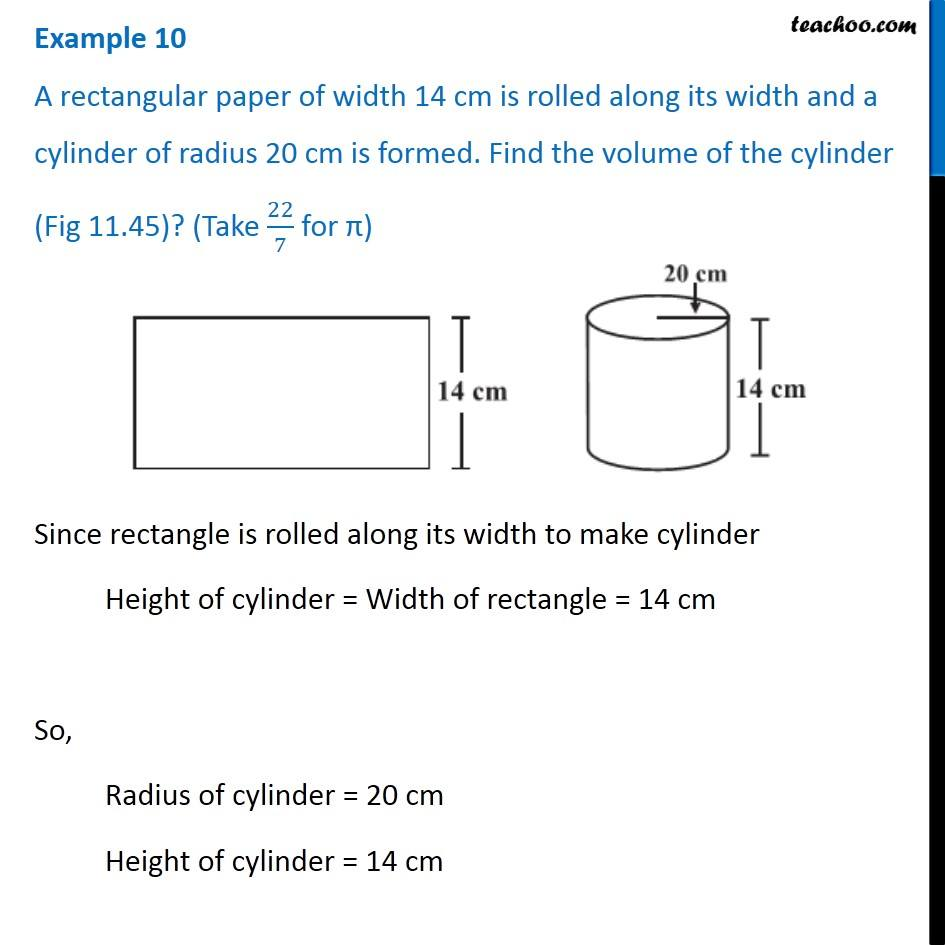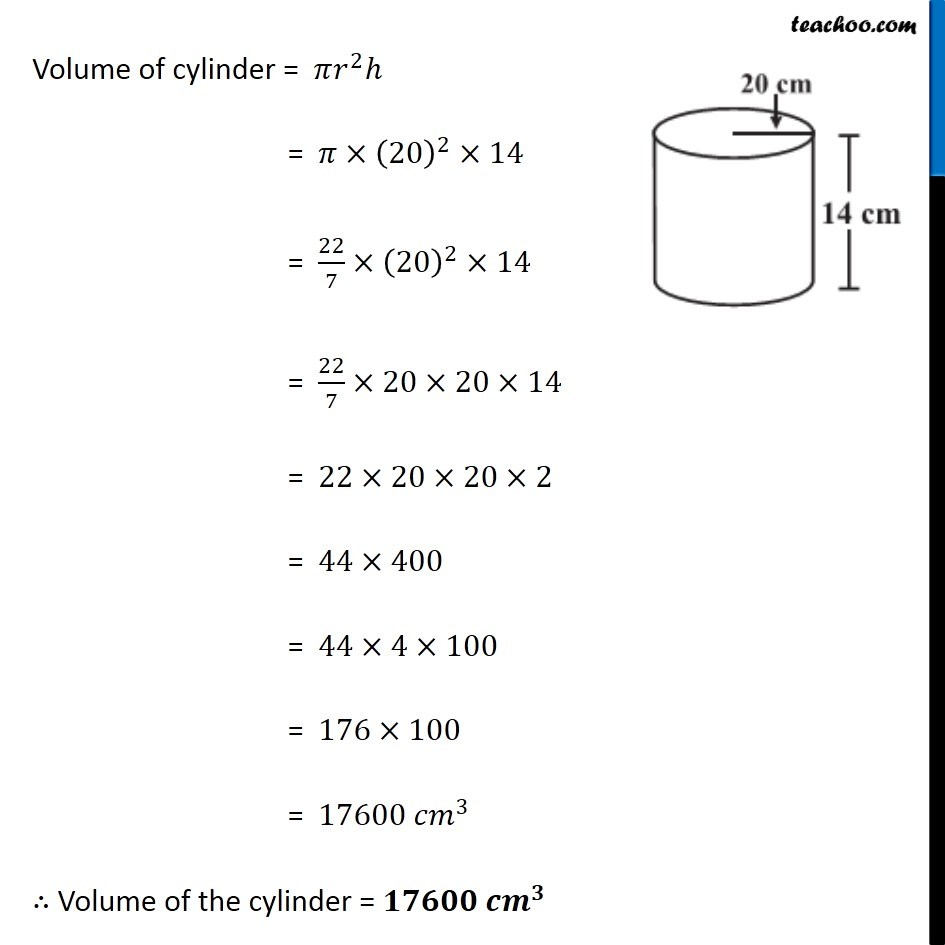Examples

Chapter 11 Class 8 Mensuration
Serial order wiseGet live Maths 1-on-1 Classs - Class 6 to 12

### Transcript

Example 10 A rectangular paper of width 14 cm is rolled along its width and a cylinder of radius 20 cm is formed. Find the volume of the cylinder (Fig 11.45)? (Take 22/7 for π) Since rectangle is rolled along its width to make cylinder Height of cylinder = Width of rectangle = 14 cm So, Radius of cylinder = 20 cm Height of cylinder = 14 cm Since rectangle is rolled along its width to make cylinder Height of cylinder = Width of rectangle = 14 cm So, Radius of cylinder = 20 cm Height of cylinder = 14 cm Volume of cylinder = 𝜋𝑟^2 ℎ = 𝜋×(20)^2×14 = 22/7×(20)^2×14 = 22/7×20×20×14 = 22×20×20×2 = 44×400 = 44×4×100 = 176×100 = 17600 〖𝑐𝑚〗^3 ∴ Volume of the cylinder = 𝟏𝟕𝟔𝟎𝟎 〖𝒄𝒎〗^𝟑